MODEL Statement

<label:> MODEL response = <effects> </ options> ;

Main effects and interaction terms can be specified in the MODEL statement, as in the GLM procedure ( Chapter 41, The GLM Procedure. ) Classification variables in the MODEL statement must be specified in the CLASS statement.

The optional label, which must be a valid SAS name, is used to label output from the matching MODEL statement.

### Options

You can specify the following options for the model fit.

CORRB

produces the estimated correlation matrix of the parameter estimates. When the resampling method is used to compute the confidence intervals, the QUANTREG procedure computes the bootstrap correlation. When the sparsity method is used to compute the confidence intervals, the procedure computes the asymptotic correlation based on an estimator of the sparsity function. The rank method for confidence intervals does not provide a correlation estimate.

COVB

produces the estimated covariance matrix of the parameter estimates. When the resampling method is used to compute the confidence intervals, the QUANTREG procedure computes the bootstrap covariance. When the sparsity method is used to compute the confidence intervals, the procedure computes the asymptotic covariance based on an estimator of the sparsity function. The rank method for confidence intervals does not provide a covariance estimate.

CUTOFF=value

specifies the multiplier of the cutoff value for outlier detection. The default value is 3.

DIAGNOSTICS<(ALL)>

requests the outlier diagnostics. By default, only observations identified as outliers or leverage points are displayed. To request that all observations be displayed, specify the ALL option.

ITPRINT

displays the iteration history of the interior point algorithm or the smoothing algorithm.

LEVERAGE<(CUTOFF=value | CUTOFFALPHA=value | H=n)>

requests an analysis of leverage points for the continuous covariates. The results are added to the diagnostics table, which you can request with the DIAGNOSTICS option in the MODEL statement. You can specify the cutoff value for leverage-point detection with the CUTOFF= option. The default cutoff value is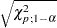, wherecan be specified with the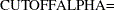option. By default,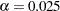. You can use the H= option to specify the number of points to be minimized for the MCD algorithm used for the leverage-point analysis. By default, H=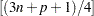, where n is the number of observations and p is the number of independent variables. The LEVERAGE option is ignored if the model includes classification variables as covariates.

NODIAG

suppresses the computation for outlier diagnostics. If you specify the NODIAG option, the diagnostics summary table will not be provided.

NOINT

specifies no intercept regression.

NOSUMMARY

suppresses the computation for summary statistics. If you specify the NOSUMMARY option, the summary statistics table will not be provided.

PLOT=plot-option
PLOTS=(plot-options)

You can use the PLOTS= option in the MODEL statement together with ODS Graphics to request the quantile process plot in addition to all plots available with the PLOT= option in the PROC statement. The plot options in the PROC statement overwrite the plot options in the MODEL statement if you specify the same options in both statements.

You can specify the following plot option only in the MODEL statement:
QUANTPLOT<(EFFECTS) </ <NOLIMITS> <EXTENDCI> <UNPACK> <OLS> > >

plots the regression quantile process. The estimated coefficient of each specified covariate effect is plotted as a function of the quantile. If you do not specify a covariate effect, quantile processes are plotted for all covariate effects in the MODEL statement. You can use the NOLIMITS option to suppress confidence bands for the quantile processes. By default, confidence bands are plotted, and process plots are displayed in panels, each of which can hold up to four plots. By default, the confidence limits are plotted for quantiles in the range between 0.05 and 0.95. You can use the EXTENDCI option to plot the confidence limits even for quantiles outside this range. You can use the UNPACK option to create individual process plots. For an individual process plot, you can superimpose the ordinary least squares estimate and its confidence limits by specifying the OLS option. The confidence level of the ordinary least squares estimate is specified with the ALPHA= option in the PROC statement.

Again, ODS Graphics must be enabled before requesting plots.

For more information about enabling and disabling ODS Graphics, see the section Enabling and Disabling ODS Graphics in Chapter 21, Statistical Graphics Using ODS.

QUANTILE=number-list | PROCESS

specifies the quantile levels for the quantile regression. You can specify any number of quantile levels in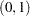. You can also compute the entire quantile process by specifying the PROCESS option. Only the simplex algorithm is available for computing the quantile process.

If you do not specify the QUANTILE= option, the QUANTREG procedure fits a median regression, which corresponds to QUANTILE=0.5.

SCALE=number

specifies the scale value used to compute the standardized residuals. By default, the scale is computed as the corrected median of absolute residuals. See the section Leverage Point and Outlier Detection for details.

SEED=number

specifies the seed for the random number generator used to compute the MCMB confidence intervals. This seed is also used to randomly select the subgroups for preprocessing when you specify the PP option in the PROC statement. If you do not specify a seed, or if you specify a value less than or equal to zero, the seed is generated from reading the time of day from the computer clock.

By default or if you specify zero, the QUANTREG procedure generates a seed between one and one billion.

SINGULAR=value

sets the tolerance for testing singularity of the information matrix and the crossproducts matrix for the initial least squares estimates. Roughly, the test requires that a pivot be at least this value times the original diagonal value. By default, SINGULAR=1E12.